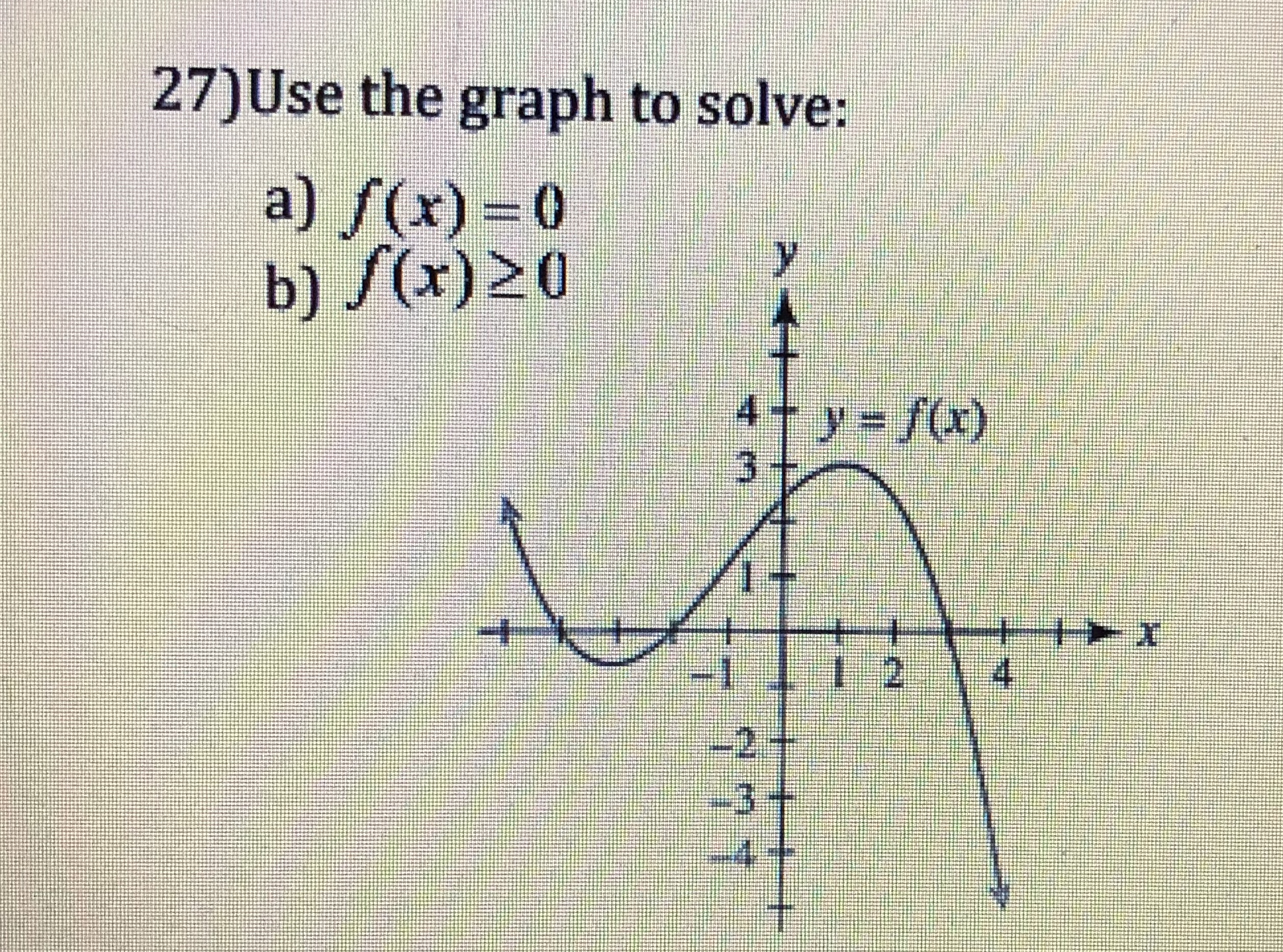Question
1 viewshelp_outlineImage Transcriptionclose27)Use the graph to solve: a)/(x) = 0 b) (x)20 4+y=f(x) 彬 fullscreen
check_circle

Step 1

a)   It is known that, y = 0 at x-axis.

From the graph, it is observed that the x-intercepts of the function y = f(x) are −4, −2, and 3.

That is, the function f(x) = 0 at the points x = −4, −2, and 3.

Step 2

b) Obtain the interval which the function f(x) greater than or equal to 0.

From part (a), the function...

### Want to see the full answer?

See Solution

#### Want to see this answer and more?

Solutions are written by subject experts who are available 24/7. Questions are typically answered within 1 hour.*

See Solution
*Response times may vary by subject and question.
Tagged in

### Other